# Angular momentum Definition , Formula ,Calculation

## Angular momentum

• In any Inertial frame of Reference the moment of linear momentum of a particle is known as angular momentum or, angular momentum of a particle is defined as the moment of its linear momentum.
• In rotational motion, angular momentum has the same significance as Linear Momentum have in the linear motion of a particle.
• Value of angular momentum of angular momentum is equal to the product of linear momentum and p(=mv) and the position vector r of the particle from origin of axis of rotation.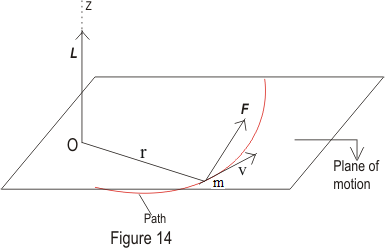• Angular momentum vector is usually represented by L.
• If the linear momentum of any particle is p=mv and its position vector from any constant point be r then angular momentum of the particle is given by
L = r×p = m(r×v)               (1)
• Angular momentum is a vector quantity and its direction is perpendicular to the direction of r and p and could be found out by right hand screw rule.
• From equation 1 scalar value or magnitude of angular momentum is given as
|L|=rpsinθ                    (2)
where V is the angle between r and p.
• For a particle moving in a circular path
v=ω×r;                    (3)
where ω is the angular velocity.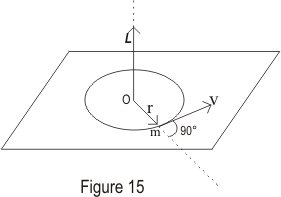Therefore
L=m[r×(ω×r)] = m{ω(r.r)-r(r.ω)} = mr2ω=Iω;                    (4)
(r.ω)=0 because in circular motion r and ω are perpendicular to each other. Here I is the moment of inertia of the particle about the given axis also the direction of L and ω is same and this is a axial vector.
writing equation 1 in the component form we get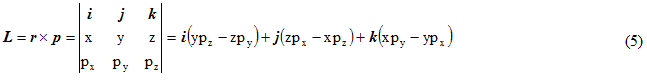• Writing angular momentum in component form we get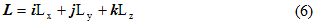writing equation 5 again we get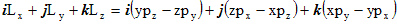Comparing unit vectors on both the sides we get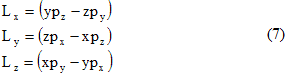• Unit of angular momentum in CGS is gm.cm2/sec and in MKS system it is Kgm.m2/sec or Joule/sec.

## Relation between angular momentum and torque

• Differentiating equation 1 w.r.t. t we get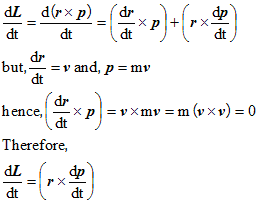• But from newton's second law of motion we have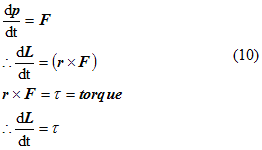Hence rate of change of angular momentum with time is equal to the torque of the force.

Question 1. A mass is whirled in a circular path with constant angular velocity and its angular momentum is L.If the string is now halve keeping the angular velocity same then angular momentum is
a. L
b. L/4
c. L/2
d. 2L
Solution 1
Angular momentum for this is defined as
=mr2ω

First case
L=mr2ω

Second case
Lf=m(r/2)2ω

So, Lf=L/4

Question 2.A mass is moving with constant velocity along a line parallel to xaxis away from origin.its angular momentum with respect to origin is
a. is zero
b. remains constant
c. goes on increasing
d. goes on decreasing

Solution 2

L=(mv)Xr
or
L=mvrsinθ
Now rsinθ=perpendicular distance from x axis which is constant
So Angular momentum is constant